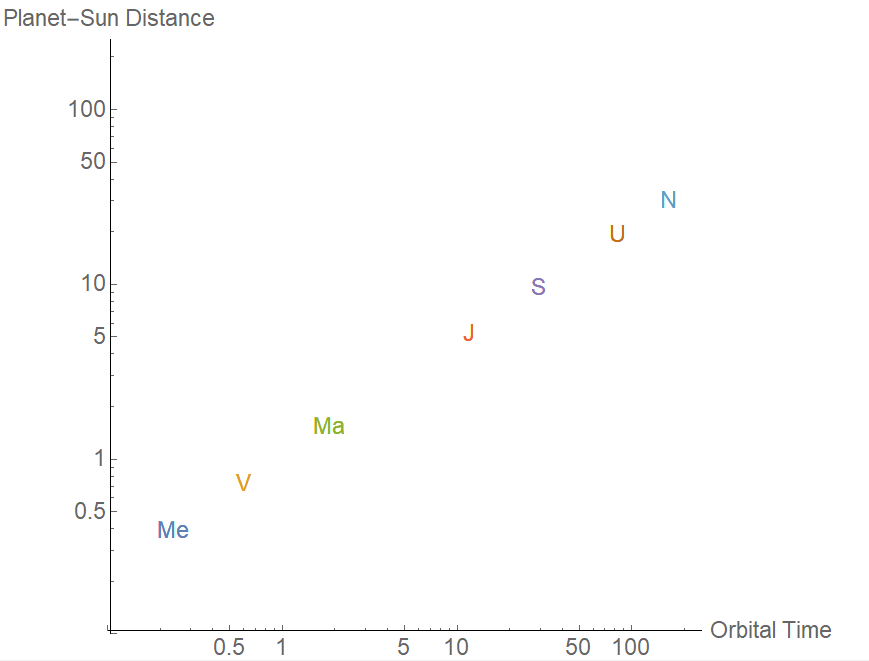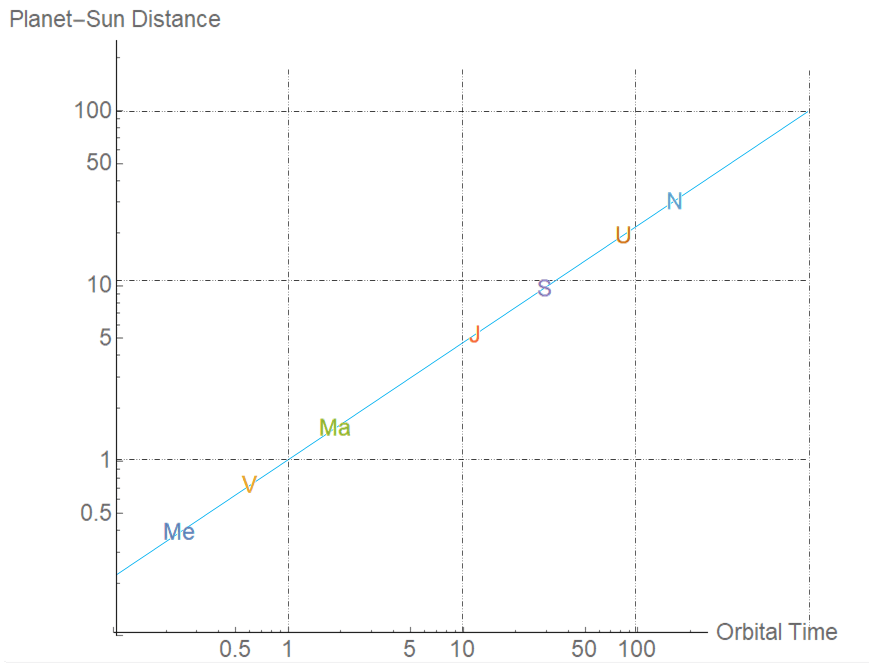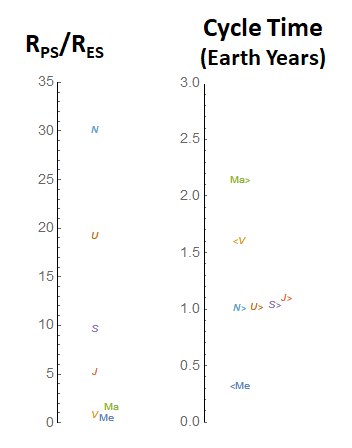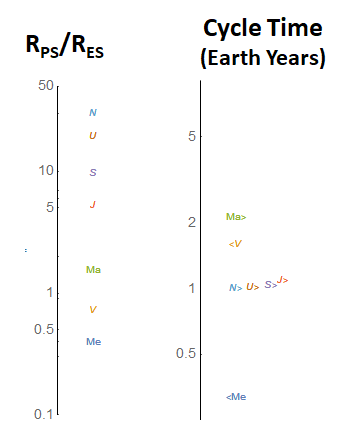## Is it Meaningful to Say that Earth Goes Round the Sun, or Not? (And Why Is This So Hard…?)

Is the statement “The Sun Orbits the Earth” false? Not according to professor Richard Muller of the University of California, Berkeley, as I discussed yesterday. Muller argues that Einstein’s theory of general relativity implies that you can view the Sun as orbiting the Earth if you like, or that both the Sun and Earth orbit Venus, or a random point in space, or anything else for that matter. Meanwhile, every science textbook in our kids’ classrooms says that “The Earth Orbits the Sun“. But for all of our discussions yesterday on this subject, we did not yet collectively come to any conclusions as to whether Muller is right or wrong. And we can’t hope to find evidence that the Earth orbits the Sun if the reverse is equally true!

When we’re trying to figure out whether a confusing statement is really true or not, we have to speak precisely. Up to this stage, I haven’t been careful enough, and in this post, I’m going to try to improve upon that. There are a few small but significant points of clarification to make first. Then we’ll look in detail at what it means to “change coordinates” in such a way that would put the Sun in orbit around the Earth, instead of the other way round.

## Sun Around the Earth, or Earth Around the Sun?  Did Einstein Say “It’s all the same”?

We’re all taught in school that the Earth goes round the Sun.  But if you look around on the internet, you will find websites that say something quite different. There you will find the argument that Einstein’s great insights imply otherwise — that in fact the statements “The Earth goes round the Sun” and “The … Read more

## From Kepler’s Law to Newton’s Gravity, Yourself — Part 2

Sometimes, when you’re doing physics, you have to make a wild guess, do a little calculating, and see how things turn out.

In a recent post, you were able to see how Kepler’s law for the planets’ motions (R3=T2 , where R the distance from a planet to the Sun in Earth-Sun distances, and T is the planet’s orbital time in Earth-years), leads to the conclusion that each planet is subject to an acceleration a toward the Sun, by an amount that follows an inverse square law

• a = (2π)2 / R2

where acceleration is measured in Earth-Sun distances and in Earth-Years.

That is, a planet at the Earth’s distance from the Sun accelerates (2π)2 Earth-distances per Earth-year per Earth-year, which in more familiar units works out (as we saw earlier) to about 6 millimeters per second per second. That’s slow in human terms; a car with that acceleration would take more than an hour to go from stationary to highway speeds.

What about the Moon’s acceleration as it orbits the Earth?  Could it be given by exactly the same formula?  No, because Kepler’s law doesn’t work for the Moon and Earth.  We can see this with just a rough estimate. The time it takes the Moon to orbit the Earth is about a month, so T is roughly 1/12 Earth-years. If Kepler’s law were right, then R=T2/3 would be 1/5 of the Earth-Sun distance. But we convinced ourselves, using the relation between a first-quarter Moon and a half Moon, that the Moon-Earth distance is less than 1/10 othe Earth-Sun distance.  So Kepler’s formula doesn’t work for the Moon around the Earth.

### A Guess

But perhaps objects that are orbiting the Earth satisfy a similar law,

• R3=T2 for Earth-orbiting objects

except that now T should be measured not in years but in Moon-orbits (27.3 days, the period of the Moon’s orbit around the Earth) and R should be measured not in Earth-Sun distances but in Moon-Earth distances?  That was Newton’s guess, in fact.

Newton had a problem though: the only object he knew that orbits the Earth was the Moon.  How could he check if this law was true? We have an advantage, living in an age of artificial satellites, which we can use to check this Kepler-like law for Earth-orbiting objects, just the way Kepler checked it for the Sun-orbiting planets.  But, still there was something else Newton knew that Kepler didn’t. Galileo had determined that all objects for which air resistance is unimportant will accelerate downward at 32 feet (9.8 meters) per second per second (which is to say that, as each second ticks by, an object’s speed will increase by 32 feet [9.8 meters] per second.) So Newton suspected that if he converted the Kepler-like law for the Moon to an acceleration, as we did for the planets last time, he could relate the acceleration of the Moon as it orbits the Earth to the acceleration of ordinary falling objects in daily life.

## From Kepler’s Law to Newton’s Gravity, Yourself — Part 1

Now that you’ve discovered Kepler’s third law — that T, the orbital time of a planet in Earth years, and R, the radius of the planet’s orbit relative to the Earth-Sun distance, are related by

• R3=T2

the question naturally arises: where does this wondrous regularity comes from?

We have been assuming that planets travel on near-circular orbits, and we’ll continue with that assumption to see what we can learn from it. So let’s look in more detail at what happens when any object, not just a planet, travels in a circle at a constant speed.

## Why Didn’t Copernicus Figure Out Kepler’s Third Law Decades Earlier?

Kepler’s third law is so simple to state that (as shown last time) it is something that any grade school kid, armed with Copernicus’s data and a calculator, can verify. Yet it was 75 years from Copernicus’s publication til Kepler discovered this formula! Why did it take Kepler until 1618, nearly 50 years of age, to recognize such a simple relationship? Were people just dumber than high-school students back then?

Here’s a clue. We take all sorts of math for granted that didn’t exist four hundred years ago, and calculations which take an instant now could easily take an hour or even all day. (Imagine computing the cube root of 4972.64 to part-per-million accuracy by hand.) In particular, one thing that did not exist in Copernicus’ time, and not even through much of Kepler’s, was the modern notion of a logarithm.

## Breakthrough! Do-it-Yourself Astronomy Pays Off: A Law of Nature (and a Bonus)

In recent posts (here and here), I showed you methods that anyone can use for estimating the planets’ distances from the Sun; it just takes high-school trigonometry. And even more recently I showed how, using just algebra, you can easily obtain the planets’ orbital periods from their cycles as we see them from Earth, starting with one solar conjunction and ending at the next.

Much of this work was done by Nicolai Copernicus himself, the most famous of those philosophers who argued for a Sun-centered universe rather than an Earth-centered universe during the millennia before modern science. He had all the ingredients we have, minus knowledge of Uranus and Neptune, and minus the clues we obtain from telescopes, which would have confirmed he was correct.

Copernicus knew, therefore, that although the planetary distances from the Sun and their cycles in the sky (which astrologers [not astronomers] have focused on for centuries) don’t seem to be related, the distances and their orbital times around the Sun are much more closely related. That’s what we saw in the last post.

Let me put these distances and times, relative to the Earth-Sun distance and the Earth year, onto a two-dimensional plot. [Here the labels are for Mercury (Me), Venus (V), Mars (Ma), Jupiter (J), Saturn (S), Uranus (U) and Neptune (N).] The first figure shows the planets out to Saturn (the ones known to Copernicus).Figure 1: The planets’ distances from the Sun (in units of the Earth-Sun distance) versus the time it takes them to complete an orbit (in Earth-years). Shown are the planets known since antiquity: Mercury (Me), Venus (V), Mars (M), Jupiter (J) and Saturn (S).

The second shows them out to Neptune, though it bunches up the inner planets to the point that you can’t really see them well.Figure 2: Same as Figure 1, but now showing Uranus (U) and Neptune (N) as well.

You can see the planets all lie along a curve that steadily bends down and to the right.

Copernicus knew all of the numbers that go into Figure 1, with pretty moderate precision. But there’s something he didn’t recognize, which becomes obvious if we use the right trick. In the last post, we sometimes used a logarithmic axis to look at the distances and the times. Now let’s replot Figure 2 using a logarithmic axis for both the distances and the times.Figure 3: Same as Figure 2, but now with both axes in logarithmic form.

Oh wow. (I’m sure that’s the equivalent of what Kepler said in 1618, when he first painstakingly calculated the equivalent of this plot.)

It looks like a straight line. Is it as straight as it looks?Figure 4: Same as Figure 3, but with a blue line added to show how well a straight line describes the distance/time relationship of the planets, and with a grid added that passes through 1, 10, 100, 1000 Earth-years and distances equal to 1, 10, 100 Earth-Sun distances.

And now we see three truly remarkable things about this graph:

• First, the planet’s distances to the Sun and orbital times lie on a very straight line on a logarithmic plot.
• Second, the slope of the line is 2/3 (2 grid steps up for every 3 steps right) rather than, say, 7.248193 .
• Third, the line goes right through the point (1,1), where the first horizontal and first vertical lines cross.

What do they mean?

## How You Can Determine Each Planet’s Year from its Distances and Cycles

Whether you’re a die-hard who insists on measuring the distances between the planets and the Sun yourself (which I’ve shown you how to do here and here), or you are willing to accept what other people tell you about them, it’s interesting to look at the pattern among these distances. They are shown at right, starting with the smallest — Mercury (Me) — and proceeding to Venus (V), Mars (Ma), Jupiter (J), Saturn (S), Uranus (U) and Neptune (N), nearly 100 times further out than Mercury. The inner planets up to Mars are very close together, all bunched within 1.5 times the Earth-Sun distance, whereas the outer planets are much further apart.Figure 1: (Left) the planet-Sun distances relative to the Earth-Sun distance; (Right) the time between solar conjunctions, when the planet disappears (roughly) behind the Sun from Earth’s perspective; “>” indicates the planet moves into the morning sky (east) after conjunction, whereas “<” means it moves into the evening sky (west).

Also shown in the figure are the lengths of the planet’s cycles. Remember, a cycle starts when a planet reappears from behind the Sun and ends when a planet again disappears behind the Sun… the moment of “solar conjunction,” or just “conjunction” for short in this post. Some planets have short cycles, others have long ones. Interestingly, now it is the outer planets that all bunch up together, with their cycles just a bit longer than an Earth year, whereas Mercury, Venus and Mars have wildly different cycles ranging from a third of an Earth-year to two Earth-years. In the figure I’m also keeping track of something that I didn’t mention before. As their cycles begin, Mercury and Venus initially move into the evening sky, in the west, setting just after sunset. I’ve indicated that with a “<” Meanwhile Mars, Jupiter and Saturn move into the eastern morning sky, rising just before sunrise, as indicated with a “>”. (Mars, Jupiter and Saturn just reappeared from behind the Sun this winter; that’s why they’re all in the morning sky right now.) This difference is going to prove important in a moment.

Before going on, let me make another version of the same figure, easier to read. This involves making a “logarithmic plot”. Instead of showing the step from 1 to 2 as the same as the step from 0 to 1, as we usually do, we replot the information so that the step along the axis from 1 to 10 is the same as the step from 0.1 to 1. It’s gives exactly the same information as the Figure 1, but now the planet-Sun distances don’t bunch up as much.

### Orbits Vs. CyclesFigure 2: exactly the same as Figure 1, but with the vertical axis in “log-plot” form.

Now, the cycles from one solar conjunction to the next, long beloved of astrologers, are not beloved of astronomers, because they involve a combination of two physically unrelated motions. A solar conjunction happens when a planet disappears behind the Sun from Earth’s perspective, so the time between one conjunction and the next combines:

• the orbital motion of the planet around the Sun;
• the yearly rotation of the line between the Sun and the Earth. (So far, we haven’t found evidence as to whether the Sun moves around the Earth or the Earth moves around the Sun — and we’ll remain agnostic about that today.)

So what astronomers want to know is the orbital period of each planet — it’s own year. That is, how long does it takes each planet to orbit the Sun, from the planet’s perspective, or from the Sun’s perspective. This is the time that an observer on the Sun would see for the planet to complete a circle relative to the fixed stars, and vice versa. (Remember we gathered evidence that the stars are fixed, or extremely slowly drifting from the perspective of the Earth, using a gyroscope, whereas either or both the Sun or the Earth are rotating relative to one another by about one degree per day. We also know the stars are much further than the Sun from our two measurements of the Moon’s radius.)